Thursday, August 6, 2020
Home > download jstse papers > JSTSE PREVIOUS YEAR QUESTION PAPER 2015-16

# JSTSE PREVIOUS YEAR QUESTION PAPER 2015-16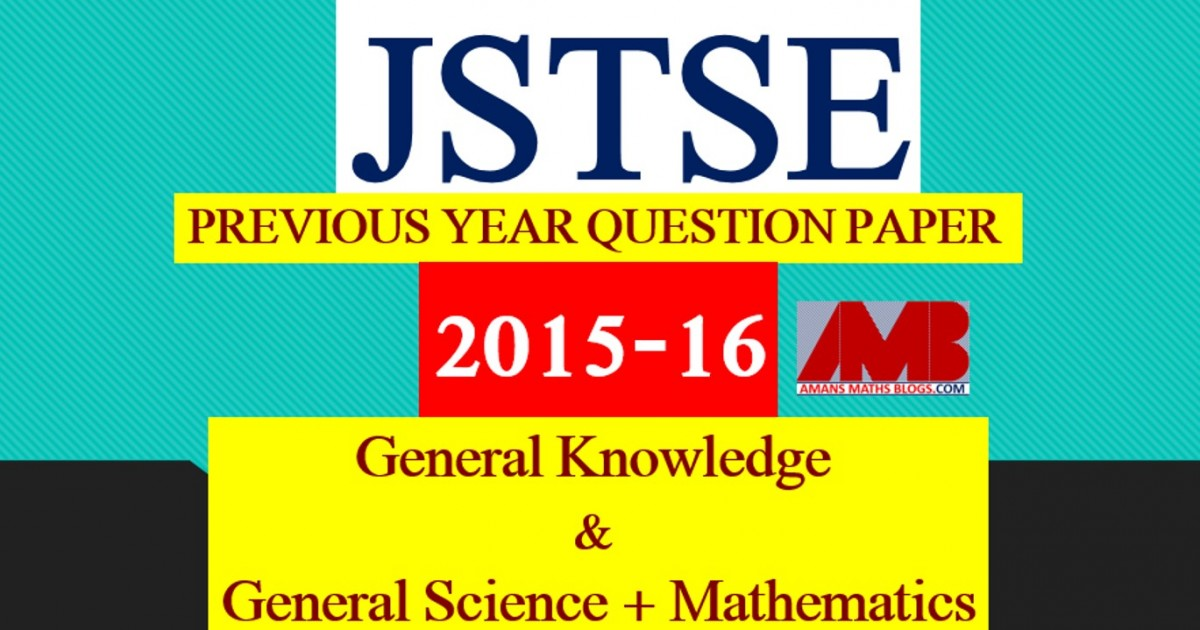# JSTSE PREVIOUS YEAR QUESTION PAPER 2015-16

Junior Science Talent Search Examination (JSTSE) is conducted by Science Branch of DTE of Education, Delhi every year usually in the month of January for the students of recognized schools of Delhi. In this post, you will get JSTSE Previous Year Question Paper 2015-16.

JSTSE
Previous Year Question Papers
2007-082008-092009-102010-112011-12
2012-132013-142014-152015-162016-17
2018-19PhysicsChemistryMathsBiology

In JSTSE, there are two papers: Paper 1 : General Knowledge and Paper 2 :  General Science & Mathematics.

The eligibility of JSTSE exam is any students of recognizing school studying in Class 9th in Govt/Public/KV/Navodaya etc and must have secured 65% marks in Class 8th. In JSTSE, there are 150 scholarships for JSTSE per year.

Read More : 129 Maths Short Tricks

## General Science + Mathematics

JSTSE Previous Year Question Paper 2015-16 Ques No 1:

If x = √6 – √3, y = √3 – √2, z = √2 – √6, then find the value of x3 + y3 + z3 – 2xyz

Options:

A. 3√2 – 4√3 + √6

B. 0

C. 3√2 + 5√3 – √6

D. √6 + √3 + √2

JSTSE Previous Year Question Papers 2015-16 Ques No 2:

The value of √(12+ √(12+ √(12+ √(12+ …))))  is

Options:

A. -3

B. 4

C. 12

D. 3

JSTSE Previous Year Question Paper 2015-16 Ques No 3:

The remainders when x45 is divided by (x2 – 1) is

Options:

A. 0

B. x

C. 2x

D. -x

JSTSE Previous Year Question Papers 2015-16 Ques No 4:

Find the value of (a + b)2/[(b – c)(c – a)] + (b + c)2/[(a – b)(c – a)] + (c + a)2/[(a – b)(b – c)].

Options:

A. 0

B. 1

C. 2

D. -1

JSTSE Previous Year Question Paper 2015-16 Ques No 5:

If √2 = 1.4142, then the value of √(√2 – 1)/(√2 + 1) is

Options:

A. 2.4142

B. 0.4142

C. 5.8282

D. 0.1718

JSTSE Previous Year Question Papers 2015-16 Ques No 6:

If x/y + y/x = -1, (where x and y are non-zeroes), then the value of (x3 – y3) is

Options:

A. 1

B. 1/2

C. 0

D. -1

JSTSE Previous Year Question Paper 2015-16 Ques No 7:

If a, b and c are all non-zero and a + b + c = 0, then the value of a2/bc + b2/ca + c2/ab is

Options:

A. 3

B. -3

C. 0

D. -1

JSTSE Previous Year Question Papers 2015-16 Ques No 8:

A person saves 180 minutes in covering a certain distance when he increases his speed form 25 km/h to 30 km/h. Find the distance.

Options:

A. 250 km

B. 420 km

C. 450 km

D. 560 km

JSTSE Previous Year Question Paper 2015-16 Ques No 9:

The greatest 5 digit number exactly divisible by 9, 13 and 17 is

Options:

A. 99945

B. 99954

C. 99450

D. 99918

JSTSE Previous Year Question Papers 2015-16 Ques No 10:

If P : Q : R = 2 : 3 : 4 and P2 + Q2 + R2 = 11600, then find the value of P – Q + R.

Options:

A. 60

B. 20

C. 24

D. 100

JSTSE Previous Year Question Paper 2015-16 Ques No 11:

In the adjoining figure, ABCD is a rectangle and E and F are the midpoints of the side BC and CD respectively. What is the ratio of the area of ∆AEF to the area of ∆FCE?Options:

A. 2 : 1

B. 5 : 2

C. 3 : 1

D. 2 : 3

JSTSE Previous Year Question Paper 2015-16 Ques No 12:

If a1/m = b1/n = c1/p and abc = 1, then the value of m + n + p is

Options:

A. 1

B. 2

C. -1

D. 0

JSTSE Previous Year Question Papers 2015-16 Ques No 13:

By selling 18 chocolates, a vendor losses the selling price of 2 chocolates. Loss percent is

Options:

A. 8%

B. 10%

C. 12%

D. 6%

JSTSE Previous Year Question Paper 2015-16 Ques No 14:

12 women can do a piece of work in 20 days. If the 4 women denied to work, then to complete the work number of more days required will be

Options:

A. 6 days

B. 10 days

C. 12 days

D. 30 days

JSTSE Previous Year Question Papers 2015-16 Ques No 15:

In figure, if BC passes through the center of the circle and AB = AC = a units, then the area of the shaded region isOptions:

A. (a2/2)(3 – π)

B. a2(π/2 – 1)

C. 2a2(π – 1)

D. (a2/2)(π/2 – 1)

JSTSE Previous Year Question Paper 2015-16 Ques No 16:

If there are two children in a family, then the probability of at least one girl in a family is

Options:

A. 1/4

B. 1/2

C. 1

D. 3/4

JSTSE Previous Year Question Papers 2015-16 Ques No 17:

The value of 0.357 is

Options:

A. 322/999

B. 322/990

C. 161/900

D. 161/450

JSTSE Previous Year Question Paper 2015-16 Ques No 18:

If (x + 1/x) = 3, then the value of (x3 + 1/x3)(x2 + 1/x2)

Options:

A. 396

B. 243

C. 126

D. 125

JSTSE Previous Year Question Papers 2015-16 Ques No 19:

The marked price of a radio is Rs. 3840. The shopkeeper allows us to discount of 10% and gains 8%. If no discount is allowed, his gains percent would be

Options:

A. 18%

B. 20%

C. 25%

D. 30%

JSTSE Previous Year Question Paper 2015-16 Ques No 20:

If the median of the observations in ascending order 24, 25, 26, (x+2), (x+3), 30, 31, 34 is 27.5, then the value of x is

Options:

A. 24

B. 25

C. 26

D. 27

JSTSE Previous Year Question Papers 2015-16 Ques No 21:

Mean of 9 observation was to be found 40. Later on, it was detected that an observation 21 was misread as 12. The correct mean is

Options:

A. 39

B. 40

C. 41

D. 42

JSTSE Previous Year Question Paper 2015-16 Ques No 22:

If volume of a cube is V units3, the surface area is A units2 and the length of a diagonal is D units. Then

Options:

C. 3A = VD

D. 6D = AV

JSTSE Previous Year Question Papers 2015-16 Ques No 23:

In the figure, there are three semi-circles. The radii of both smaller semi-circles is equal. If the radius of the larger semi-circle is 22 cm, then the area of shaded region is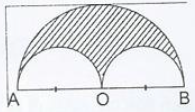Options:

A. 363π/4 cm2

B. 121π cm2

C. 236.5π cm2

D. 363π cm2

JSTSE Previous Year Question Paper 2015-16 Ques No 24:

The value of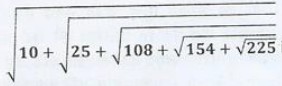Options:

A. 2

B. 3

C. 4

D. 5

JSTSE Previous Year Question Papers 2015-16 Ques No 25:

In the given figure, ∠AQB is equal to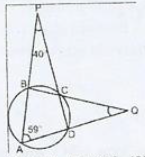Options:

A. 18 degree

B. 20 degree

C. 22 degree

D. 24 degree

JSTSE Previous Year Question Paper 2015-16 Ques No 26:

Factors of (x6 – 1) are

Options:

A. (x + 1)(x – 1)(x4 + x2 + 1)

B. (x + 1)(x – 1)(x4 – x2 – 1)

C. (x + 1)(x – 1)(x2 + 1)(x2 – 1)

D. (x + 1)(x – 1)(x4 + x3 + x2 + x + 1)

JSTSE Previous Year Question Papers 2015-16 Ques No 27:

How many sides does a regular polygon have if the measure of an exterior angle is 24 degree?

Options:

A. 12

B. 13

C. 15

D. 18

JSTSE Previous Year Question Papers 2015-16 Ques No 28:

In the figure, ABCD is a rhombus. AC and BD are its diagonals intersecting at O. If ∠OAB = 37 degree, then find the ∠OCD + ∠OBA is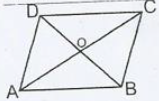Options:

A. 90 degree

B. 143 degree

C. 43 degree

D. 127 degree

JSTSE Previous Year Question Papers 2015-16 Ques No 29:

A hollow sphere with internal and external radius as 7 cm and 8 cm respectively is melted and recast into a cone with a height of 16 cm. The diameter of the base of the cone will be

Options:

A. 26 cm

B. 6.5 cm

C. 13 cm

D. 10 cm

JSTSE Previous Year Question Papers 2015-16 Ques No 30:

In the given figure, AB || CD, value of x is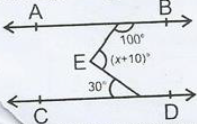Options:

A. 100 degree

B. 90 degree

C. 110 degree

D. 140  degree

JSTSE
Previous Year Question Papers
2007-082008-092009-102010-112011-12
2012-132013-142014-152015-162016-17
2018-19PhysicsChemistryMathsBiology
error: Content is protected !!